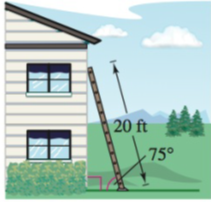Chapter 8.2, Problem 71E### Calculus: An Applied Approach (Min...

10th Edition
Ron Larson
ISBN: 9781305860919

#### Solutions

Chapter
Section### Calculus: An Applied Approach (Min...

10th Edition
Ron Larson
ISBN: 9781305860919
Textbook Problem
1 views

# Length A 20-foot ladder leaning against the side of a house makes a 75° angle with the ground (see figure). How far up the side of the house does the ladder reach?To determine

To calculate: The height of the house where ladder reaches, the length of the ladder is 20-foot and the angle between ground and ladder is 75°.

Explanation

Given Information:

The provided figure is shown below:

The length of the ladder is 20-foot and the angle between ground and ladder is 75°.

Formula used:

The definition of trigonometric function Sine.

sinθ=yr

Where, x is the length of the side adjacent to θ, y is the length of the side opposite to θ, and r=x2+y2 is the length of hypotenuse which is not equal to 0.

Calculation:

Consider the provided figure is,

From the figure, the side BC is 20 ft and angle between the side AB and BC is 75° where BC is the length of hypotenuse and AB is the length of the side adjacent of θ, and AC is the length of the side opposite to θ

### Still sussing out bartleby?

Check out a sample textbook solution.

See a sample solution

#### The Solution to Your Study Problems

Bartleby provides explanations to thousands of textbook problems written by our experts, many with advanced degrees!

Get Started

#### Sketch the graphs of the equations in Exercises 512. x+y=1

Finite Mathematics and Applied Calculus (MindTap Course List)

#### In problems 23-58, perform the indicated operations and simplify. 43.

Mathematical Applications for the Management, Life, and Social Sciences

#### Evaluate the integral. 8. t2sintdt

Single Variable Calculus: Early Transcendentals

#### What is the partial fraction form of ?

Study Guide for Stewart's Single Variable Calculus: Early Transcendentals, 8th